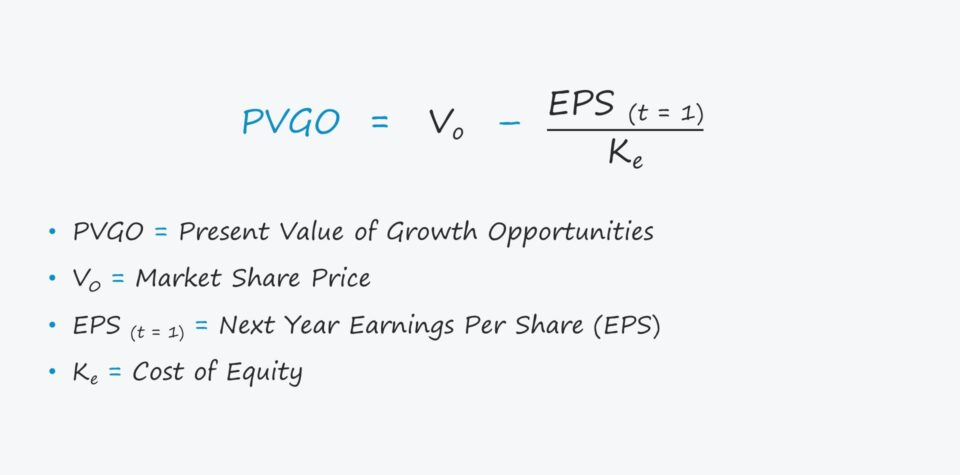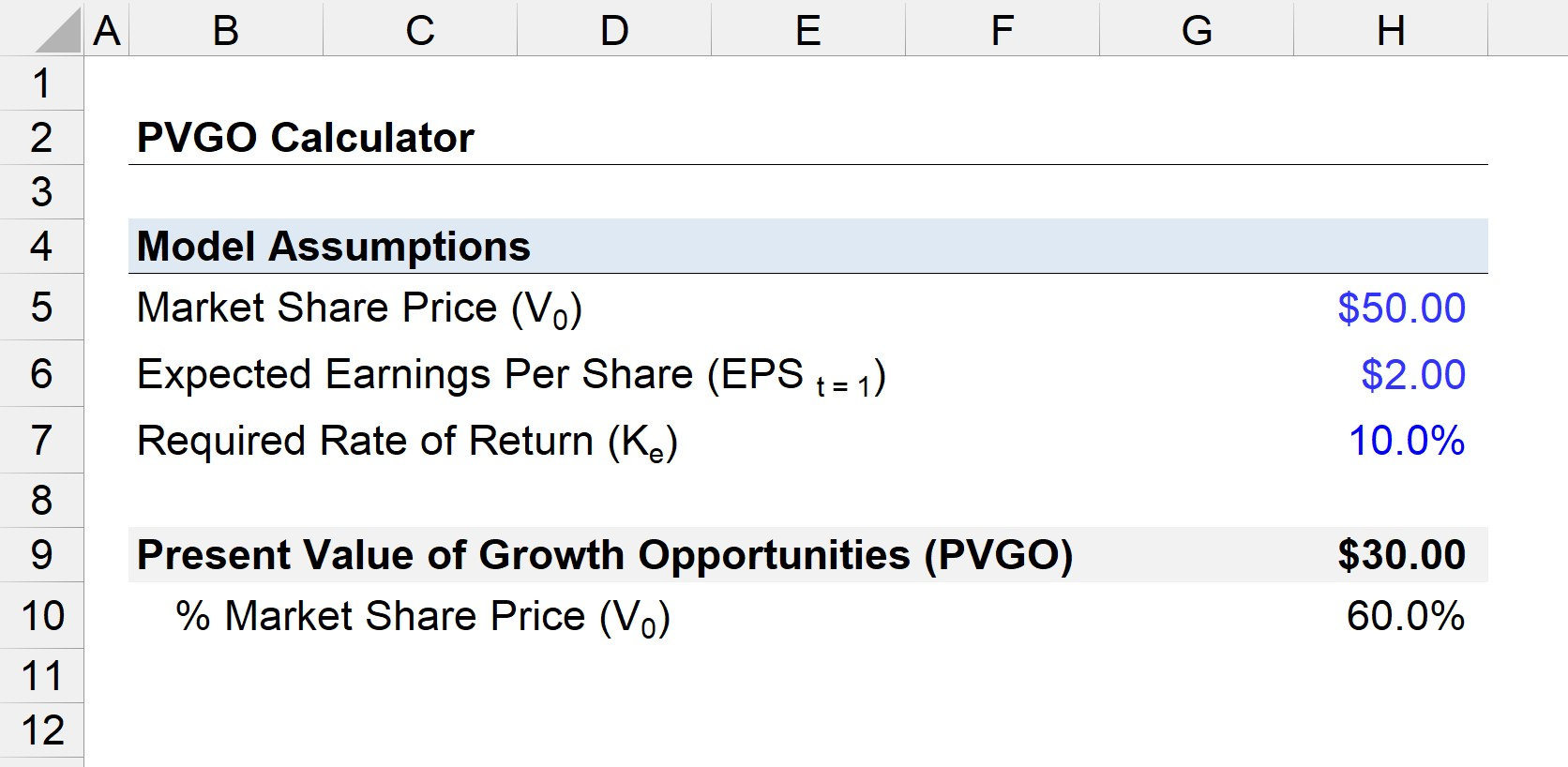# Present Value of Growth Opportunities (PVGO)

Guide to Understanding the Present Value of Growth Opportunities (PVGO)• What is the definition of the present value of growth opportunities (PVGO)?
• What is the formula for calculating the present value of growth opportunities (PVGO)?
• How is the present value of growth opportunities (PVGO) calculated?
• Why does the present value of growth opportunities (PVGO) matter?

## Present Value of Growth Opportunities (PVGO) Definition

PVGO is the component of a company’s share price corresponding to expectations of future earnings growth.

PVGO, shorthand for the “present value of growth opportunities,” represents the value of a company’s future growth.

The PVGO metric measures the potential value-creation from a company reinvesting earnings back into itself, i.e. from accepting projects to drive future growth.

There are two components to a company’s current share price:

1. Present Value (PV) of No-Growth Earnings
2. Present Value (PV) of Earnings with Growth

The earnings with no growth can be valued as a perpetuity, where the expected earnings per share (EPS) next year is divided by the cost of equity (Ke).

The latter part, the future earnings growth, is what PVGO attempts to measure, i.e. the value of growth.

## PVGO Formula

The formula shown below states that a company’s valuation is equal to the sum of the present value (PV) of its no-growth earnings and the present value of growth opportunities (PVGO).

###### Market Share Price Formula
• Vo = [EPS (t =1) / Ke ] + PVGO

Where:

After rearranging the formula, the PVGO formula is as follows.

###### PVGO Formula
• PVGO = Vo – [EPS (t =1) / Ke]

Therefore, PVGO is conceptually the difference between a company’s value minus the present value (PV) of its earnings, assuming zero growth.

## Interpreting PVGO — Reinvest or Payout Dividends?

The higher the PVGO, the more earnings should be invested rather than issuing dividends to shareholders (and vice versa).

In theory, the objective of all corporates should be to maximize shareholder wealth.

That being said, shareholder wealth is created when companies consistently reinvest earnings into positive net present value (NPV) projects.

If there are no projects worth pursuing from a returns standpoint, these zero-growth companies should distribute their earnings to shareholders in the form of dividends.

More specifically, a negative PVGO implies that by reinvesting earnings, a company is eroding value rather than creating it.

Hence, a negative PVGO company should distribute more of its net earnings to shareholders as dividends.

But if a company’s PVGO is positive — i.e. ROE is greater than its cost of capital — reinvesting into future growth can generate more value for shareholders than dividend payments.

An industry-leading PVGO suggests the company has far more growth opportunities in its pipeline that could be pursued than its peers, which results in greater upside potential in a company’s future share price.

The PVGO can be a useful guide in the critical decision-making process of picking between reinvesting earnings or paying out dividends.

• If PVGO < 0 → Distribute Earnings as Dividends
• If PVGO > 0 → Reinvest Earnings

PVGO is often expressed as a percentage of the current market share price (Vo).

• High PVGO % of Vo → Greater Present Value (PV) Contribution from Growth Expectations
• Low PVGO % of Vo → Lower Present Value (PV) Contribution from Growth Expectations
###### Normalized Share Price

One limitation to PVGO is the assumption that the current share price reflects the Company’s fair value, which can be quite a risky assertion considering how volatile (and irrational) the market can be.

Thus, it is recommended to either ensure the share price is normalized to reflect historical performance or use the one-year average share price.

## PVGO Calculator — Excel Template

We’ll now move to a modeling exercise, which you can access by filling out the form below.Submitting ...

## PVGO Example Calculation

Suppose a company is currently trading at a share price of \$50.00, with the market anticipating its earnings per share (EPS) next year to be \$2.00.

If we assume a required rate of return of 10%, what proportion of the company’s market price is attributable to its future growth?

• Market Share Price (Vo) = \$50.00
• Expected Earnings Per Share (EPS t=1) = \$2.00
• Cost of Equity (Ke) = 10%

After entering the provided assumption into our share price formula from earlier, we are left with the following:

• \$50.00 = (\$2.00 / 10%) + PVGO

By dividing the EPS expected next year by the required rate of return (i.e. the cost of equity), we arrive at the zero-growth valuation of \$20.

We can now solve for PVGO by rearranging the formula and then subtracting the zero-growth valuation price component (\$2.00 / 10% = \$20.00) from the total valuation.

• \$50.00 = \$20.00 + PVGO
• PVGO = \$50.00 – \$20.00 = \$30.00

Upon dividing the \$30 PVGO by the \$50 share price, we can conclude that the market assigns 60% of the market price to future growth — which implies that significant growth expectations are priced into our illustrative company’s current share price.

• PVGO % Vo = \$30.00 / \$50.00 = 60%Step-by-Step Online Course

#### Everything You Need To Master Financial Modeling

Enroll in The Premium Package: Learn Financial Statement Modeling, DCF, M&A, LBO and Comps. The same training program used at top investment banks.Inline FeedbacksLearn Financial Modeling Online

Everything you need to master financial and valuation modeling: 3-Statement Modeling, DCF, Comps, M&A and LBO.

X

The Wall Street Prep Quicklesson Series

7 Free Financial Modeling Lessons

Get instant access to video lessons taught by experienced investment bankers. Learn financial statement modeling, DCF, M&A, LBO, Comps and Excel shortcuts.